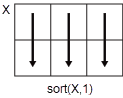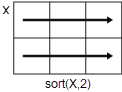# sort

Sort elements of symbolic arrays

## Syntax

``Y = sort(X)``
``Y = sort(X,dim)``
``Y = sort(___,direction)``
``````[Y,I] = sort(___)``````

## Description

example

````Y = sort(X)` sorts the elements of `X` in ascending lexicographical order. If `X` is a vector, then `sort(X)` sorts the vector elements of `X`.If `X` is a matrix, then `sort(X)` treats the columns of `X` as vectors and sorts each column independently.If `X` is a multidimensional array, then `sort(X)` operates along the first array dimension whose size does not equal 1, treating the elements as vectors. ```

example

````Y = sort(X,dim)` sorts the elements of `X` along the dimension `dim`. For example, if `X` is a two-dimensional matrix, then `sort(X,1)` sorts the elements of each column of `X`, and `sort(X,2)` sorts the elements of each row.```

example

````Y = sort(___,direction)` returns sorted elements of `X` in the order specified by `direction` using any of the previous syntaxes. `'ascend'` indicates ascending order (the default), and `'descend'` indicates descending order.```

example

``````[Y,I] = sort(___)``` also returns a collection of index vectors for any of the previous syntaxes. `I` is the same size as `X` and describes the arrangement of the elements of `X` into `Y` along the sorted dimension. For example, if `X` is an `m`-by-`n` matrix and you sort the elements of each column (`dim = 1`), then each column of `I` is an index vector of the sorted column of `X`, such that for j = 1:n Y(:,j) = X(I(:,j),j); end ```

## Examples

collapse all

Create a symbolic row vector and sort its elements in ascending order.

```syms a b c d e sort([7 e 1 c 5 d a b])```
`ans = $\left(\begin{array}{cccccccc}1& 5& 7& a& b& c& d& e\end{array}\right)$`

When sorting the elements of a matrix, `sort` can work along the columns or rows of that matrix.

Create a symbolic matrix.

`X = magic(3)*sym('a')`
```X =  $\left(\begin{array}{ccc}8 a& a& 6 a\\ 3 a& 5 a& 7 a\\ 4 a& 9 a& 2 a\end{array}\right)$```

Sort the matrix `X`. By default, the `sort` command sorts the elements of each column.

`Y = sort(X)`
```Y =  $\left(\begin{array}{ccc}3 a& a& 2 a\\ 4 a& 5 a& 6 a\\ 8 a& 9 a& 7 a\end{array}\right)$```

To sort the elements of each row, set the value of the `dim` option to `2`.

`Y = sort(X,2)`
```Y =  $\left(\begin{array}{ccc}a& 6 a& 8 a\\ 3 a& 5 a& 7 a\\ 2 a& 4 a& 9 a\end{array}\right)$```

Create a symbolic matrix.

`X = magic(3)*sym('a')`
```X =  $\left(\begin{array}{ccc}8 a& a& 6 a\\ 3 a& 5 a& 7 a\\ 4 a& 9 a& 2 a\end{array}\right)$```

Sort the elements of each row in descending order.

`Y = sort(X,2,'descend')`
```Y =  $\left(\begin{array}{ccc}8 a& 6 a& a\\ 7 a& 5 a& 3 a\\ 9 a& 4 a& 2 a\end{array}\right)$```

To find the indices that each element of a matrix `Y` had in the original matrix `X`, call `sort` with two output arguments.

Create a symbolic matrix `X`.

`X = magic(3)*sym('a')`
```X =  $\left(\begin{array}{ccc}8 a& a& 6 a\\ 3 a& 5 a& 7 a\\ 4 a& 9 a& 2 a\end{array}\right)$```

Sort each column of `X` and return the indices of the sorted elements in `I`. Each column of `I` contains the presorted positions of entries in `Y`.

`[Y,I] = sort(X)`
```Y =  $\left(\begin{array}{ccc}3 a& a& 2 a\\ 4 a& 5 a& 6 a\\ 8 a& 9 a& 7 a\end{array}\right)$```
```I = 3×3 2 1 3 3 2 1 1 3 2 ```

Sort a symbolic vector `X` that contains real and complex numbers. When `X` contains symbolic real and complex numbers, `sort(X)` returns the sorted real numbers, followed by the sorted complex numbers based on their real parts.

`X = sort(sym([2 -1/2 3+4i 5i 4+3i]))`
```X =  $\left(\begin{array}{ccccc}-\frac{1}{2}& 2& 5 \mathrm{i}& 3+4 \mathrm{i}& 4+3 \mathrm{i}\end{array}\right)$```

Create a 2-by-2-by-2 symbolic array that contains symbolic numbers, variables, and functions.

```syms x y f(x); X(:,:,1) = [y 1; 1/3 x]; X(:,:,2) = [x -2; 1/4 f(x)]; X```
```X(:,:,1) =  $\left(\begin{array}{cc}y& 1\\ \frac{1}{3}& x\end{array}\right)$```
```X(:,:,2) =  $\left(\begin{array}{cc}x& -2\\ \frac{1}{4}& f\left(x\right)\end{array}\right)$```

Sort its elements in ascending order along the third dimension.

`Y = sort(X,3)`
```Y(:,:,1) =  $\left(\begin{array}{cc}x& -2\\ \frac{1}{4}& x\end{array}\right)$```
```Y(:,:,2) =  $\left(\begin{array}{cc}y& 1\\ \frac{1}{3}& f\left(x\right)\end{array}\right)$```

Use `X(:)`, the column representation of `X`, to sort all of the elements of `X`.

`Y = sort(X(:))`
```Y =  $\left(\begin{array}{c}-2\\ \frac{1}{4}\\ \frac{1}{3}\\ 1\\ x\\ x\\ y\\ f\left(x\right)\end{array}\right)$```

## Input Arguments

collapse all

Input array, specified as a symbolic vector, matrix, or multidimensional array. `sort` uses the following rules:

• If `X` contains only symbolic real numbers that are rational, then `sort(X)` sorts the elements numerically.

• If `X` contains only symbolic complex numbers with rational real and imaginary parts, then `sort(X)` sorts the elements first by their real parts, then by their imaginary parts to break ties.

• If `X` contains only symbolic variables, then `sort(X)` sorts the elements alphabetically.

• If `X` contains a mix of symbolic numbers (with rational parts) and variables, then `sort(X)` returns the following sequence: sorted real numbers, sorted complex numbers, and sorted variables.

• If `X` contains symbolic irrational numbers, expressions, and functions, comparing and sorting the elements can be computationally complex. Therefore, `sort` uses internal sorting rules to optimize its performance.

Dimension to operate along, specified as a positive integer scalar. If no value is specified, then the default is the first array dimension whose size does not equal 1.

Consider a two-dimensional input array `X`:

• `sort(X,1)` sorts the elements in the columns of `X`.• `sort(X,2)` sorts the elements in the rows of `X`.`sort` returns `X` if `dim` is greater than `ndims(X)`.

Sorting direction, specified as `'ascend'` or `'descend'`.

## Output Arguments

collapse all

Sorted array, returned as a symbolic vector, matrix, or multidimensional array. `Y` is the same size and type as `X`.

Sort index, returned as a symbolic vector, matrix, or multidimensional array. `I` is the same size as `X`. The index vectors are oriented along the same dimension that `sort` operates on. For example, if `X` is a 2-by-3 matrix, then ```[Y,I] = sort(X,2)``` sorts the elements in each row of `X`. The output `I` is a collection of 1-by-3 row index vectors that contains the presorted positions of each row of `Y`.

## Tips

• Calling `sort` for arrays of numbers that are not symbolic objects invokes the MATLAB® `sort` function.

• The `sort` function sorts symbolic complex numbers differently from MATLAB floating-point complex numbers. For symbolic input `X` that contains complex numbers, `sort(X)` sorts the complex numbers first by their real parts, then by their imaginary parts to break ties. For floating-point input `X`, by default, `sort(X)` sorts complex numbers by their magnitude, followed by their phase angles in the interval (−π, π] to break ties.

## Version History

Introduced before R2006a

expand all